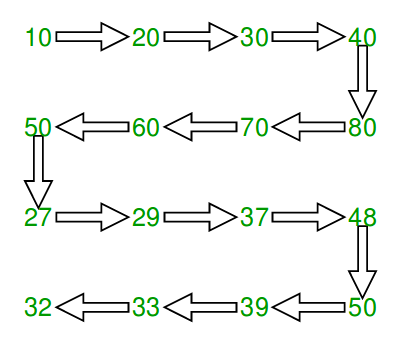Open in App
Not now

# Java Program to Print matrix in snake pattern

• Last Updated : 07 Aug, 2022

Given an n x n matrix .In the given matrix, you have to print the elements of the matrix in the snake pattern.

Examples :

```Input :mat[][] = { {10, 20, 30, 40},
{15, 25, 35, 45},
{27, 29, 37, 48},
{32, 33, 39, 50}};

Output : 10 20 30 40 45 35 25 15 27 29
37 48 50 39 33 32

Input :mat[][] = { {1, 2, 3},
{4, 5, 6},
{7, 8, 9}};
Output : 1 2 3 6 5 4 7 8 9```We traverse all rows. For every row, we check if it is even or odd. If even, we print from left to right else print from right to left.

## Java

 `// Java program to print matrix in snake order` `import` `java.util.*;` `class` `GFG` `{` `    ``static` `void` `print(``int` `[][] mat)` `    ``{` `        ``// Traverse through all rows` `        ``for` `(``int` `i = ``0``; i < mat.length; i++)` `        ``{`   `            ``// If current row is even, print from` `            ``// left to right` `            ``if` `(i % ``2` `== ``0``)` `            ``{` `                ``for` `(``int` `j = ``0``; j < mat[``0``].length; j++)` `                    ``System.out.print(mat[i][j] +``" "``);`     `            ``// If current row is odd, print from` `            ``// right to left` `            ``}` `            ``else` `            ``{` `                ``for` `(``int` `j = mat[``0``].length - ``1``; j >= ``0``; j--)` `                    ``System.out.print(mat[i][j] +``" "``);` `            ``}` `        ``}` `    ``}`   `    ``// Driver code` `    ``public` `static` `void` `main(String[] args)` `    ``{` `        ``int` `mat[][] = ``new` `int``[][]` `        ``{` `            ``{ ``10``, ``20``, ``30``, ``40` `},` `            ``{ ``15``, ``25``, ``35``, ``45` `},` `            ``{ ``27``, ``29``, ``37``, ``48` `},` `            ``{ ``32``, ``33``, ``39``, ``50` `}` `        ``};`   `        ``print(mat);` `    ``}` `}` `/* This code is contributed by Mr. Somesh Awasthi */`

Output :

`10 20 30 40 45 35 25 15 27 29 37 48 50 39 33 32 `

Time complexity: O(n^2) where n is no of elements in rows and columns

Auxiliary Space: O(1) because constant space has been used

Please refer complete article on Print matrix in snake pattern for more details!

My Personal Notes arrow_drop_up
Related Articles